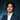# Autoencoders in SAS Viya

June 09, 2020

One of the critical steps when doing data preparation for modeling is feature extraction. Feature extraction allows to get informative data as an input to Machine Learning models. Dimensionality reduction techniques are used in feature extraction as a mean to compress the information and extract less, but more meaningful features for the models.

These are some of the main methods in the toolset of Data Scientists:

• Principal Component Analysis (PCA)
• Singular Value Decomposition (SVD)
• Autoencoder

We will focus here on Autoencoder. These steps were performed on SAS Viya 3.5

## Autoencoder

Autoencoder is an unsupervised learning technique from Neural Networks. When designing the neural network, we force the network to go through a compressed representation of the input (encoder). This representation is then used to reconstruct the input (decoder). By doing so we train the neural network to learn a reduced representation of the data that minimizes the noise.

There are several methods to use Autoencoder in SAS Viya, we will look into two of them:

1. through the graphical interface
2. by code, using CAS actions and the Python API

## Model Studio

The first method allows to leverage the power of Autoencoders without writing a single line of code. By using the visual interface users can use Preprocessing nodes to access this feature.

In Model Studio (Pipeline View):

• Select Feature Extraction

• Autoencoder
• Automatic
• PCA
• Robust PCA
• SVD

• Set Autoencoder options

This allows to specify the architecture of the autoencoder: users can specify the number of hidden layers (3 or 5), the number of neurons in the different layers as well as the activation function. The autoencoder is trained using stochastic gradient descent (SGD), and more strategies like early stopping, weight decay and dropout can be set in the optimization options.

More details about these parameters can be found in the documentation.

## Python API

Now, we will look into implementing an autoencoder by code, using the Python API for SAS Viya.

The first step is to connect to a SAS Viya Server with your credentials.

import swat

# Connect to CAS server
s = swat.CAS('hostname.com', 5570, username='username', password='password')

Then, we need to read the data, we will use here a credit card dataset from Kaggle.

castbl = s.read_csv('creditcard.csv', casout='CREDITCARD')

To access the Autoencoder CAS Action (equivalent of functions in other languages), we need to import the neural networks actionset.

s.loadactionset('neuralNet')
• Now, we can use the artificial neural network CAS action annTrain, to train the autoencoder. We specify the following parameters:
• table: the name of the table
• inputs: the input variables
• casout: name of the output model
• arch: architecture family of the neural network
• hiddens: the hidden layers, this is a tuple with the number of neurons for each layer, example {5,3,5}
• acts: activation function
• std: standardization strategy
• nloOpts: options for the optimization
s.neuralNet.annTrain(table = 'CREDITCARD',
inputs   = [e for e in castbl.columns if e != 'Class'],
casOut = dict(name = 'nn_model', replace = True),
arch='mlp', hiddens={5}, acts={'tanh'}, std='midrange',
nloOpts={'algorithm':'lbfgs', 'optmlOpt':{'maxIters':100, 'fConv':1E-10}})

By not omitting the target parameters, and describing the hidden layers architecture, we force the annTrain CAS action to train an autoencoder.

Check the annTrain documentation for an exhaustive list of the parameters.

Now, we would like to get back the middle layer containing our compressed representation, to do so we will score the input data with the trained autoencoder.

s.neuralNet.annScore(table = 'CREDITCARD',
modelTable={'name':'nn_model'},
casOut={'name':'autoencoder_score', 'replace':'true'},
listNode='hidden',
encodeName=True)

By specifying listNode='hidden' we ask the CAS action to return the hidden layer nodes, and we are done !